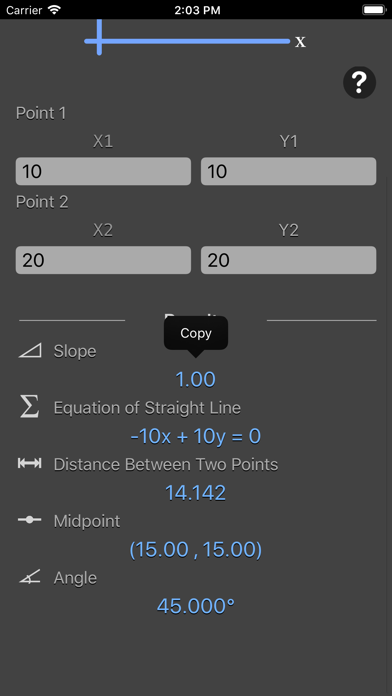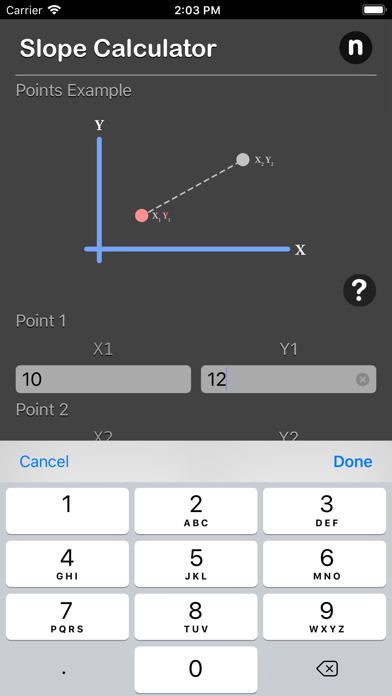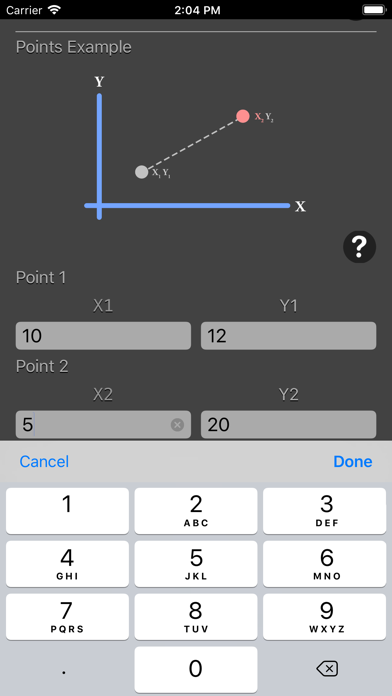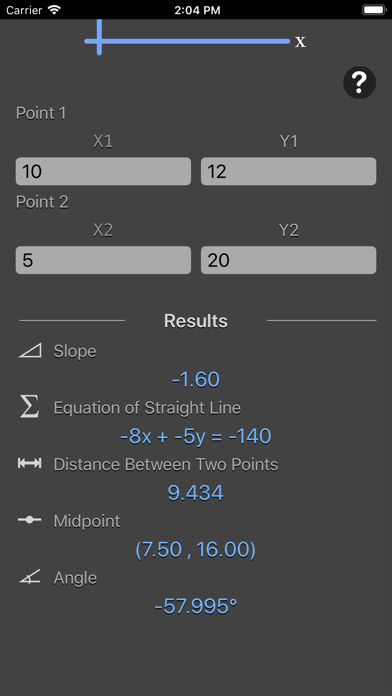## Slope Calculator Plus - Lumos Educational App Store11
Price -\$2.99
\$2.99

#### DESCRIPTION:

Slope calculator calculate slope [m], equation of straight line, distance between 2 points, midpoint and angle by input position of 2 points both on x-axis and y-axis. Features: - Calculate slope [m], equation of straight line, distance between 2 points, midpoint and angle by input position of 2 points both on x-axis and y-axis - Instant result output - Result are copy-able - Reference image for better understanding on input point - Formula are include for better understanding Slope, sometimes referred to as gradient in mathematics, is a number that measures the steepness and direction of a

#### OVERVIEW:

Slope Calculator Plus is a free educational mobile app By Nitrio.It helps students practice the following standards .

This page not only allows students and teachers download Slope Calculator Plus but also find engaging Sample Questions, Videos, Pins, Worksheets, Books related to the following topics.

Developer: Nitrio

Developer URL: http://www.nitrio.com

Software Version: 1.0

Category: Utilities

Release Date: 2018-04-30T19:52:07Z#### Are you the Developer?EdSearch WebSearch